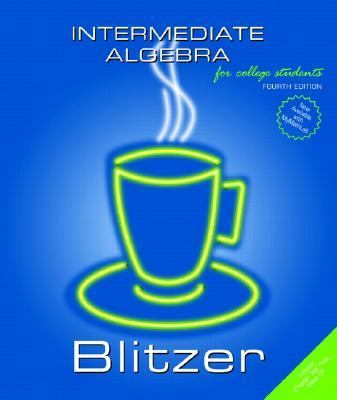2128483

9780131492578

# Intermediate Algebra for College Students\$3.33
\$3.95 Shipping
List Price
\$138.67
Discount
97% Off
You Save
\$135.34

• Condition: Good
• Provider: YourOnlineBookstore Contact
• Provider Rating:
88%
• Ships From: Houston, TX
• Shipping: Standard, Expedited30-day money back guarantee

Most renters respond to questions in 48 hours or less.
The response will be emailed to you.
• ISBN-13: 9780131492578
• ISBN: 0131492578
• Edition: 4
• Publication Date: 2005
• Publisher: Prentice Hall

### AUTHOR

Blitzer, Robert F.

### SUMMARY

CONTENTS A Brief Guide to Getting the Most from This Book Preface To The Student About the Author Chapter 1: Algebra, Mathematical Models, and Problem Solving 1.1 Algebraic Expressions and Real Numbers 1.2 Operations with Real Numbers and Simplifying Algebraic Expressions 1.3 Graphing Equations 1.4 Solving Linear Equations Mid-Chapter Check Point 1.5 Problem Solving and Using Formulas 1.6 Problems of Integral Exponents 1.7 Scientific Notation Group Project Summary Review Exercises Chapter Test Chapter 2: Functions and Linear Functions 2.1 Introduction to Functions 2.2 The Algebra of Functions Mid-Chapter Check Point 2.3 Linear Functions and Slope 2.4 The Point-Slope Form of the Equation of a Line Group Project Summary Review Exercises Chapter Test Cumulative Review Exercises (Chapters 1-2) Chapter 3: Systems of Linear Equations 3.1 Systems of Linear Equations in Two Variables 3.2 Problem Solving and Business Applications Using Systems of Equations 3.3 Systems of Linear Equations in Three Variables Mid-Chapter Check point 3.4 Matrix Solutions to Linear Systems 3.5 Determinants and Cramer's Rule Group Project Summary Review Exercises Chapter Test Chapter 4: Inequalities and Problem Solving 4.1 Solving Linear Inequalities 4.2 Compound Inequalities 4.3 Equations and Inequalities Involving Absolute Value Mid-Chapter Check Point 4.4 Linear Inequalities in Two Variables 4.5 Linear Programming Group Project Summary Review Exercises Chapter Test Cumulative Review Exercises (Chapters 1-4) Chapter 5: Polynomials, Polynomial Functions, and Factoring 5.1 Introduction to Polynomials and Polynomial Functions 5.2 Multiplication of Polynomials 5.3 Greatest Common Factors and Factoring By Grouping 5.4 Factoring Trinomials Mid-Chapter Check Point 5.5 Factoring Special Forms 5.6 A General Factoring Strategy 5.7 Polynomial Equations and Their Applications Group Project Summary Review Exercises Chapter Test Chapter 6: Rational Expressions, Functions, and Equations 6.1 Rational Expressions and Functions: Multiplying and Dividing 6.2 Adding and Subtracting Rational Expressions 6.3 Complex Rational Expressions 6.4 Division of Polynomials Mid-Chapter Check Point 6.5 Synthetic Division and the Remainder Theorem 6.6 Rational Equations 6.7 Formulas and Applications of Rational Equations 6.8 Modeling Using Variation Group Project Summary Review Exercises Chapter Test Cumulative Review Exercises (Chapters 1-6) Chapter 7: Radicals, Radical Functions, and Rational Exponents 7.1 Radical Expressions and Functions 7.2 Rational Exponents 7.3 Multiplying and Simplifying Radical Expressions 7.4 Adding, Subtracting, and Dividing Radical Expressions Mid-Chapter Check Point 7.5 Multiplying with More Than One Term and RationalizingBlitzer, Robert F. is the author of 'Intermediate Algebra for College Students ', published 2005 under ISBN 9780131492578 and ISBN 0131492578.

You can find lots of answers to common customer questions in our FAQs

View a detailed breakdown of our shipping prices